Dynamic responce from DPZTFFC device.
Consider the laminar flow of incompressible liquid between two plates, one of them is transversaly oscillating: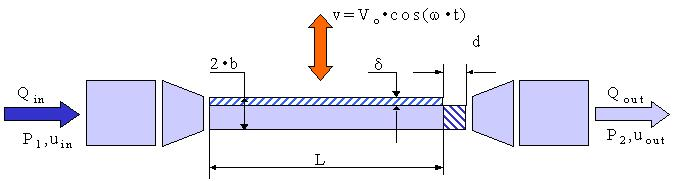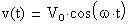From Bernoulli equation (energy conservation):when Du<<uFrom Continuity equation:and finallywhere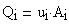Account that the liquid is incompressible let's calculate the speed changes during the superseding phase:
Hence:where :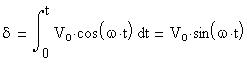andThen we can calculate the
device characteristics
(resistance to flow and
capacitance of flow):
Resistance = Potential/Flow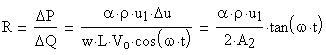Capacitance = Storage/PotentialLet's compute both parameters:where :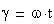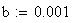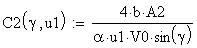2
From Bernoulli equation (energy conservation):From Continuity equation:Account that the liquid is incompressible let's calculate the speed changes during the superseding phase: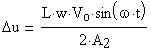Then:
Resistance = Potential/Flow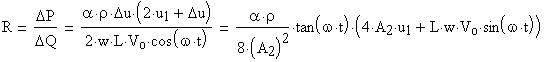Capacitance = Storage/PotentialLet's compute both parameters: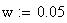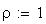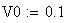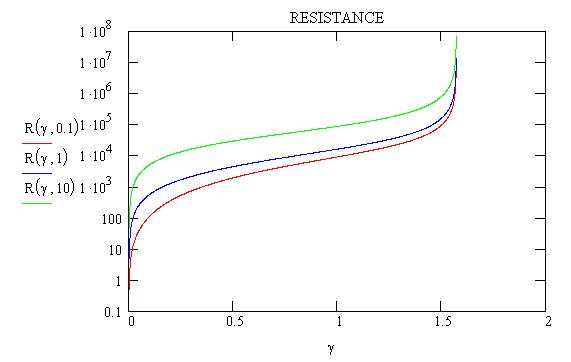Developed by Roman N Tunkel,
Research Engineer, Ph.D.,
tunkelrn@utrc.utc.com